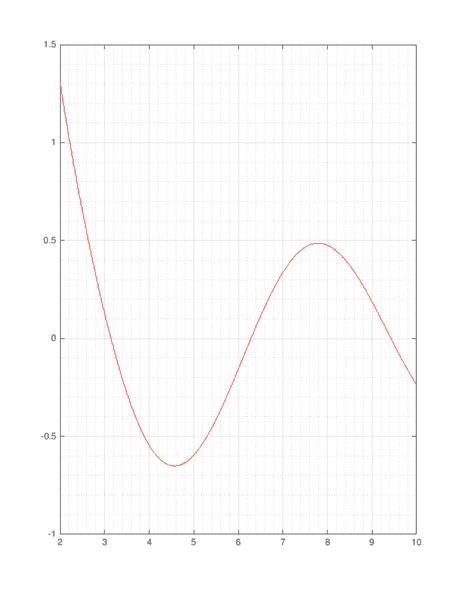# Plot of ##\sin(x)/\log(x)##.

• MATLAB
• MathematicalPhysicist

#### MathematicalPhysicist

Gold Member
Hi I want to plot the function ##y=\sin(x)/\log(x)## in MATLAB but I get the next error message:
Matlab:
Warning: Imaginary parts of complex X and/or Y arguments ignored

Here is the script that I used:
Matlab:
x = -2*pi:pi/100:2*pi;
y = sin(x)/log(x);
plot(x,y)
How to fix this that I'll get the plot on the real line?

Thanks!

Never mind.

I want to delete this thread but I don't see how...

What happens to the denominator when ##x=1##?

@kuruman Yeah, it's not defined there, then how plot this function?
when I type it in google I get a nice plot.

•MathematicalPhysicist
@kuruman Yeah, it's not defined there, then how plot this function?
when I type it in google I get a nice plot.
Can you do a piecewise plot? (I am not familiar with MATLAB).

@jedishrfu when I try to plot for the domain [2,10] I still don't get a graph, I do get the plot window but without the graph line, it doesn't show up.

Matlab:
x = 2:1/100:10;
y = sin(x)/log(x);
plot(x,y)

@jedishrfu when I try to plot for the domain [2,10] I still don't get a graph, I do get the plot window but without the graph line, it doesn't show up.
Use this:
Matlab:
y = sin(x)./log(x);
The thing I added is "./" so that ##y## becomes a matrix. Now you should get a good plot.#### Attachments

•MathematicalPhysicist and jedishrfu
the dot before the operator says to do an element by element evaluation.

as an example:

x=0:0.1:10
y=x.*x

generates the y=x^2 function and this plots it

plot(x,y)

In other programming languages, this simple convenience would be implemented as a for loop

Python:
for i in range(0, 100):
x[i] = i / 10.0

for i in range(0, 100):
y[i] = x[i] * x[i]

Ah, yes forgot about the dot thing. Thanks folks!

as an example:

x=0:0.1:10
y=x.*x

generates the y=x^2 function
Note that the dot also works for exponential operators:
Code:
y = x.^2